# How To Display A Calendar In Python

35

In this tutorial, you will learn how to display a calendar in Python. To display a calendar in Python, we can use an in-built module named calendar. Python calendar module offers many methods to work with dates and calendars.

We can use it to display a whole year calendar, a specific month calendar, or even get the day of a week for a specific date.

Apart from that, we will explore the following Python programs:

• Display a calendar of a specific year
• Display a calendar of a specific month
• Find the name of a month from a number
• Get current year
• calendar.weekday() method
• Get the day of the week for a specific date
• Calendar in HTML
• Fetch a specific day for a whole year

Also ReadHow To Sort a List of Tuples in Python

## Python Program to Display a Calendar of a Specific Year

• We will first import the Python calendar module using this statement: `import calendar`
• After that, we will define the year variable and initialize it with the value whose calendar we would like to display.
• In this final step, we will use the calendar.calendar(year) function to display the calendar.

Here’s the code for the Python program to display a calendar of a specific year.

Code:

```import calendar
year = 3000
print(calendar.calendar(year))```

Output: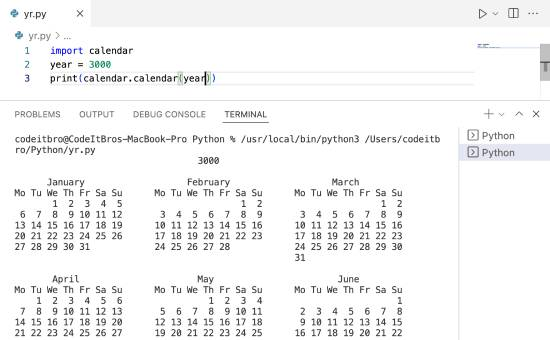Also ReadHow to Create Python Empty Set

## Calendar of a Specific Month

Now, we will see how to display a calendar of a specific month in Python.

• Again, we will import the calendar module as we did in the previous example.
• We will initialize two variables with year and month values.
• In the final step, we will display the calendar using the calendar.month(year, month) method.

Here’s the Python code to display a calendar of a specific month.

Code:

```import calendar
year = 2022
month = 5
print(calendar.month(year, month))```

Output: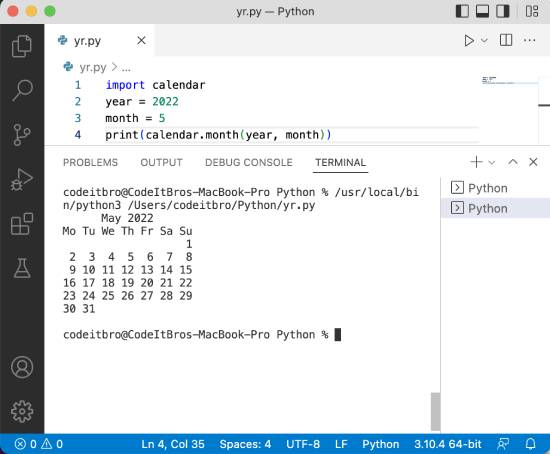## Find the Name of a Month From a Number

In this Python program, we will see how to find the name of a month from a number. For example, for the number 6, we should get the name of the sixth month (June) in the output.

• Import Python calendar module.
• Use the calendar.month_name[value] to get the month’s name.

Also ReadHow to Exit Python Program [4 Methods]

Here’s the code for your reference.

Code:

```import calendar
print(calendar.month_name)```

Output: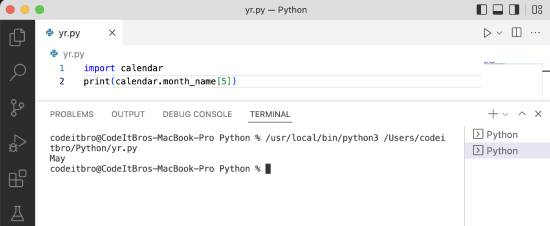Also ReadHow to Convert Python Tuples to Lists

## Get Current Year

In this example, we will use the datetime Python module to get the current year from the local system.

• Import datetime module using this statement: `import datetime`
• Initialize the CurrentYear variable with the current year’s value using the datetime.datetime.now().year function.
• Display the CurrentYear variable using the print() statement.

Code:

```import datetime
CurrentYear = datetime.datetime.now().year
print("The current year is", CurrentYear)```

Output: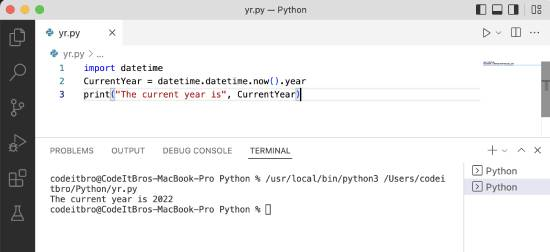Also ReadPython For Loop Index With Examples

## Python calendar.weekday() Method

You can use the calendar.weekday(year, month, day) method to get the day of the week. 0 is for Monday, 1 is for Tuesday, and so forth.

• Import Python calendar module.
• Initialize the WeekDay variable to get the current weekday using the calendar.weekday(year, month, day) function.
• Display the current weekday using the print statement.

Here’s the code for your reference.

Code:

```import calendar
WeekDay = calendar.weekday(2022, 5, 11)
print("The current week day is", WeekDay+1)```

Output: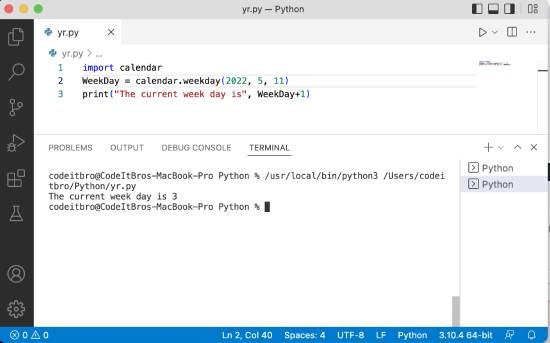## Get the Day of the Week for a Specific Date

In this Python example, we will see how to get the day of the week for a specific date. For this, we will use the calendar.weekday function to get the current weekday and then calendar.day_name[argument] to print day of the week.

• Import calendar Python package.
• Initialize WeekDayName with calendar.weekday(year, month, day) function to get the current weekday.
• Print the day of the specific date by using the calendar.day_name[WeekDayName] function.

Here’s the code of the Python program to get the day of the week for a specific date.

Code:

```import calendar
WeekDayName = calendar.weekday(2022, 5, 11)
print("The day for the specific date is", calendar.day_name[WeekDayName])```

Output:## Display an HTML calendar in Python

Here we will see how to display an HTML calendar in Python.

• Import Python calendar module.
• Initialize CalendarHTML variable using the calendar.HTMLCalendar.
• Define the CalYear variable and initialize it with the HTML code of a specific calendar year using the CalendarHTML.formatyear(year) method.
• Print the HTML calendar using the print(CalYear) statement.

Code:

```import calendar

CalendarHTML = calendar.HTMLCalendar()
CalYear = CalendarHTML.formatyear(2022)
print(CalYear)```

Output: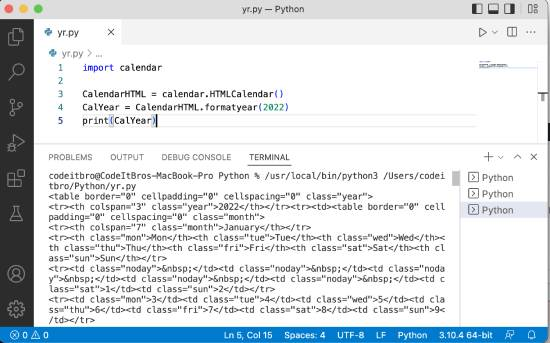## Fetch a Specific Day for a Whole Year

In this Python calendar example, we will see how to get a specific day for a whole year.

Code:

```import calendar

for month in range(1, 13):
CalYear = calendar.monthcalendar(2022, month)

FirstWeek = CalYear
SecondWeek = CalYear

if FirstWeek[calendar.TUESDAY] != 0:
InspectionDay = FirstWeek[calendar.TUESDAY]
else:
InspectionDay = SecondWeek[calendar.TUESDAY]
print("%10s %2d" % (calendar.month_name[month], InspectionDay))```

Output: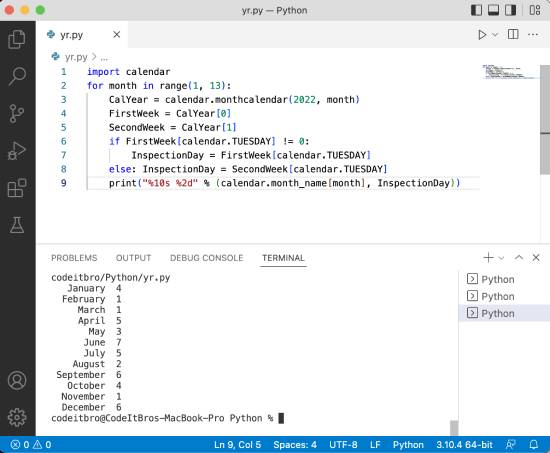### Summary

In this Python tutorial, we explored how to display a calendar in Python. You also learned how to display an HTML calendar, display a calendar of a specific month, get the current year, and many other examples related to the Python calendar module. Subscribe to our newsletter to receive such articles straight to your inbox.